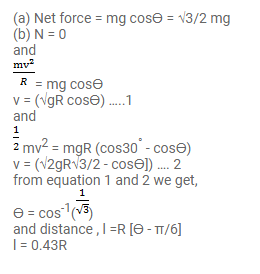# A particle of mass m is kept on a fixed,Question:

A particle of mass $\mathrm{m}$ is kept on a fixed, smooth sphere of radius $\mathrm{R}$ at a position, where the radius through the particle makes an angle of $30^{\circ}$ with the vertical. The particle is released from this position. (a) What is the force exerted by the sphere on the particle just after the release? (b) Find the distance travelled by the particle before it leaves contact with the sphere.

Solution: